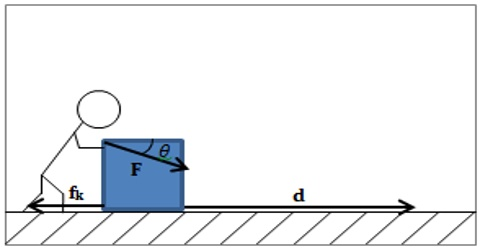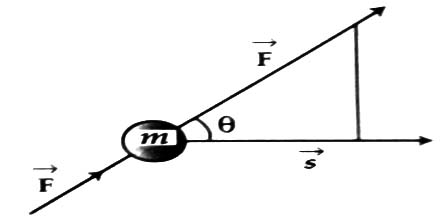Physics

# Workless ForceWorkless Force

If component of displacement along the direction of force is considered instead of considering applied force along displacement, then according to figure,

W = Fs cos θ

If expressed in vector form, we get

W = F. s

So we can say that scalar product of force and displacement is work done.From general definition of work it is seen that even if force acts but displacement of the point of action of force s = 0, then work done W = 0. Again, if displacement of the point of action of the force is perpendicular i.e., θ = 90°, then W = Fs cos 90° = 0.

That means, one or more forces can act on a body perpendicular to the direction of motion. If point of action of these forces makes an angle of 90° with the direction of displacement, then these forces do not perform any work. This type of force is called workless force.